Search Results (12)

View
Selected filters:
• CCSS.Math.Content.4.NBT.A.2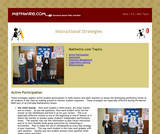Conditions of Use:
Read the Fine Print
Rating

These strategies support active student participation in math lessons and allow teachers to assess the developing proficiency levels of all students in the class by walking around to monitor student responses. These strategies are especially effective during the Mental Math part of an Everyday Mathematics lesson.

Material Type:
Teaching/Learning Strategy
Provider:
Mathwire
Author:
Terry Kawas
02/16/2011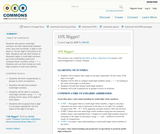Conditions of Use:
Remix and Share
Rating

Students will explore multi-digit numbers and the relationship between ones, tens and hundreds; a digit in one place is 10x the digit in the place to its right. Students will use their bodies to represent digits in multi-digit numbers up to the hundredths place and compare these numbers using <, =, >. Students will use their bodies as multi-digit numbers to add and subtract.

Subject:
Mathematics
Material Type:
Activity/Lab
Teaching/Learning Strategy
Author:
01/28/2016
Remix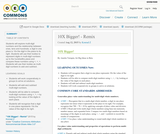Conditions of Use:
Remix and Share
Rating

Students will explore multi-digit numbers and the relationship between ones, tens and hundreds; a digit in one place is 10x the digit in the place to its right. Students will use their bodies to represent digits in multi-digit numbers up to the hundredths place and compare these numbers using <, =, >. Students will use their bodies as multi-digit numbers to add and subtract.

Subject:
Mathematics
Material Type:
Activity/Lab
Teaching/Learning Strategy
Author:
08/08/2019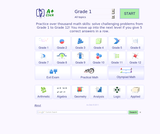Conditions of Use:
Remix and Share
Rating

A+ Click is an interactive collection of more than 3700 math problems and answers for K-1 K-12 school program. It defines the personal level of math knowledge. You move up into the next level if you give 5 correct answers in a row. Practice makes perfect.

Subject:
Mathematics
Algebra
Geometry
Material Type:
Activity/Lab
Assessment
Game
Lecture Notes
Provider:
aplusclick
NSDL Staff
Provider Set:
Individual Authors
Mathematics Gateways and Resources
Author:
Igor Kokcharov
02/16/2011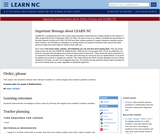Conditions of Use:
Remix and Share
Rating

This lesson lets students interact with rational numbers to create largest and smallest possible numbers.

Subject:
Mathematics
Material Type:
Lesson Plan
Provider:
University of North Carolina at Chapel Hill School of Education
Provider Set:
LEARN NC Lesson Plans
Author:
Gail Poteat
06/25/1999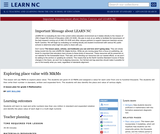Conditions of Use:
Remix and Share
Rating

This lesson will use M&Ms to explore place value. The students are given 8-10 M&Ms and assigned a value for each color from one to hundred thousand. The students will then write their number in standard, written and expanded form. The students will also identify the place and value of various digits.

A lesson plan for grade 4 Mathematics.

Subject:
Mathematics
Material Type:
Lesson Plan
Provider:
University of North Carolina at Chapel Hill School of Education
Provider Set:
LEARN NC Lesson Plans
Author:
Amy Bullins
09/19/2000
Remix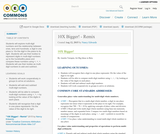Conditions of Use:
Remix and Share
Rating

Students will explore multi-digit numbers and the relationship between ones, tens and hundreds; a digit in one place is 10x the digit in the place to its right. Students will use their bodies to represent digits in multi-digit numbers up to the hundredths place and compare these numbers using <, =, >. Students will use their bodies as multi-digit numbers to add and subtract.

Subject:
Mathematics
Material Type:
Activity/Lab
Teaching/Learning Strategy
Author:
Nancy Edwards
08/08/2019
Remix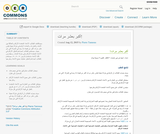Conditions of Use:
No Strings Attached
Rating

سيستكشف الطلاب الأعداد المتعددة الأرقام والعلاقة بين الآحاد والعشرات والمئات؛ الرقم في منزلة معينة يكون عشر مرات أكبر من قيمته إذا وجد في المنزلة التي عن يمينه. سيستخدم الطلاب أجسادهم لتمثيل الأرقام في الأعداد المتعددة الأرقام حتى منزلة المئات.
وسيقارنون تلك الأعداد باستخدام الرموز < ، = ، >. سيستخدم الطلاب أجسادهم لتمثيل عمليات جمع الأعداد وطرحها.

Subject:
Mathematics
Material Type:
Activity/Lab
Teaching/Learning Strategy
Author:
Pierre Tannous
08/08/2019
Remix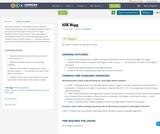Conditions of Use:
Remix and Share
Rating

Students will explore multi-digit numbers and the relationship between ones, tens and hundreds; a digit in one place is 10x the digit in the place to its right. Students will use their bodies to represent digits in multi-digit numbers up to the hundredths place and compare these numbers using <, =, >. Students will use their bodies as multi-digit numbers to add and subtract.

Subject:
Mathematics
Material Type:
Activity/Lab
Teaching/Learning Strategy
Author:
Jody Bauer
08/08/2019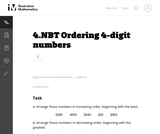Conditions of Use:
No Strings Attached
Rating

In this task students must place 4-digit numbers into order from smallest to greatest and greatest to smallest.

Subject:
Mathematics
Numbers and Operations
Material Type:
Activity/Lab
Provider:
Illustrative Mathematics
Provider Set:
Illustrative Mathematics
Author:
Illustrative Mathematics
05/01/2012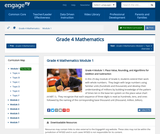Conditions of Use:
Remix and Share
Rating

In this 25-day module of Grade 4, students extend their work with whole numbers.  They begin with large numbers using familiar units (hundreds and thousands) and develop their understanding of millions by building knowledge of the pattern of times ten in the base ten system on the place value chart (4.NBT.1).  They recognize that each sequence of three digits is read as hundreds, tens, and ones followed by the naming of the corresponding base thousand unit (thousand, million, billion).

Subject:
Numbers and Operations
Material Type:
Module
Provider:
New York State Education Department
Provider Set:
EngageNY
05/11/2013
Remix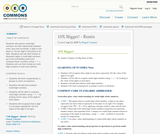Conditions of Use:
Remix and Share
Rating

Students will explore multi-digit numbers and the relationship between ones, tens and hundreds; a digit in one place is 10x the digit in the place to its right. Students will use their bodies to represent digits in multi-digit numbers up to the hundredths place and compare these numbers using <, =, >. Students will use their bodies as multi-digit numbers to add and subtract.

Subject:
Mathematics
Material Type:
Activity/Lab
Teaching/Learning Strategy
Author:
Raquel Vazquez